# High School Math : How to find the area of a square

## Example Questions

← Previous 1

### Example Question #81 : Quadrilaterals

ABCD and EFGH are squares such that the perimeter of ABCD is 3 times that of EFGH. If the area of EFGH is 25, what is the area of ABCD?

25

5

225

15

75

225

Explanation:

Assign variables such that

One side of ABCD = a

and One side of EFGH = e

Note that all sides are the same in a square. Since the perimeter is the sum of all sides, according to the question:

4a = 3 x 4e = 12e or a = 3e

From that area of EFGH is 25,

e x e = 25 so e = 5

Substitute a = 3e so a = 15

We aren’t done. Since we were asked for the area of ABCD, this is a x a = 225.

### Example Question #82 : Quadrilaterals

A square has an area of 36. If all sides are doubled in value, what is the new area?

72

108

132

48

144

144

Explanation:

Let S be the original side length. S*S would represent the original area. Doubling the side length would give you 2S*2S, simplifying to 4*(S*S), giving a new area of 4x the original, or 144.

### Example Question #83 : Quadrilaterals

If the perimeter of a square is equal to twice its area, what is the length of one of its sides?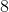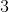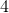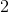Explanation:

Area of a square in terms of each of its sides:

Area = S x S

Perimeter of a square:

Perimeter = 4S

So if 'the perimeter of a square is equal to twice its area':

2 x Area = Perimeter

2 x [S x S] = [4S]; divide by 2:

S x S = 2S; divide by S:

S = 2

### Example Question #84 : Quadrilaterals

Freddie is building a square pen for his pig. He plans to buy x feet of fencing to build the pen. This will result in a pen with an area of p square feet. Unfortunately, he only has enough money to buy one third of the planned amount of fencing. Which expression represents the area of the pen he can build with this limited amount of fencing?

p/9

3p

p/6

9p

p/3

p/9

Explanation:

If Freddie uses x feet of fencing makes a square, each side must be x/4 feet long. The area of this square is (x/4)2 = x2/16 = p square feet.

If Freddie uses one third of x feet of fencing makes a square, each side must be x/12 feet long. The area of this square is (x/12)2x2/144 = 1/9(x2/16) = 1/9(p) = p/9 square feet.

Alternate method:

The scale factor between the small perimeter and the larger perimeter = 1 : 3. Since we're comparing area, a two-dimensional measurement, we can square the scale factor and see that the ratio of the areas is 1: 32 = 1 : 9.

### Example Question #85 : Quadrilaterals

If the diagonal of a square measures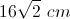, what is the area of the square?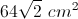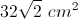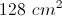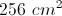Explanation:

This is an isosceles right triangle, so the diagonal must equal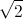times the length of a side. Thus, one side of the square measures, and the area is equal to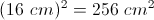### Example Question #211 : Geometry

A square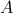has side lengths of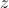. A second square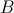has side lengths of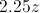. How many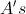can you fit in a single?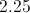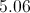Explanation:

The area ofis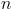, the area ofis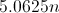. Therefore, you can fit 5.06in.

### Example Question #212 : Geometry

The perimeter of a square is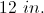If the square is enlarged by a factor of three, what is the new area?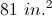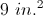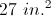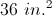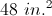Explanation:

The perimeter of a square is given by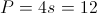so the side length of the original square is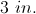The side of the new square is enlarged by a factor of 3 to give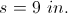So the area of the new square is given by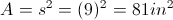.

### Example Question #36 : Squares

How much more area does a square with a side of 2r have than a circle with a radius r?  Approximate  π by using 22/7.

1/7 square units

6/7 square units

12/14 square units

4/7 square units

6/7 square units

Explanation:

The area of a circle is given by A = πr2 or 22/7r2

The area of a square is given by A = s2 or (2r)2 = 4r2

Then subtract the area of the circle from the area of the square and get 6/7 square units.

### Example Question #37 : Squares

If the perimeter of a square is 44 centimeters, what is the area of the square in square centimeters?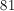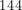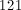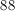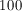Explanation:

Since the square's perimeter is 44, then each side is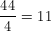.

Then in order to find the area, use the definition that the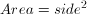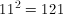### Example Question #38 : Squares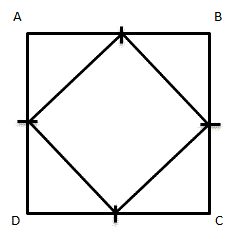Given square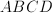, with midpoints on each side connected to form a new, smaller square.  How many times bigger is the area of the larger square than the smaller square?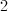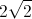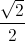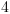Explanation:

Assume that the length of each midpoint is 1.  This means that the length of each side of the large square is 2, so the area of the larger square is 4 square units.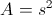To find the area of the smaller square, first find the length of each side.  Because the length of each midpoint is 1, each side of the smaller square is(use either the Pythagorean Theorem or notice that these right trianges are isoceles right trianges, so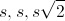can be used).

The area then of the smaller square is 2 square units.

Comparing the area of the two squares, the larger square is 2 times larger than the smaller square.

← Previous 1